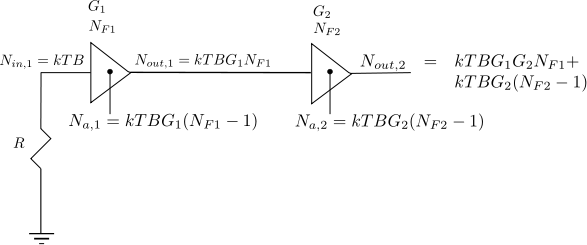- DSP log - http://www.dsplog.com -

Posted By Krishna Sankar On April 21, 2012 @ 7:38 am In Analog | 4 Comments

Following the discussion on thermal noise and it’s modeling  and noise figure computation for a simple resistor network , in this article let us discuss the Noise Figure of cascaded stages.

## Definition of Noise Figure

From Section 2.3.2 of RF Microelectronics, Behzad Razavi, the noise figure is defined as

, where

is the input signal to noise ratio and

is the output signal to noise ratio.

Consider that the system has a gain  and is the noise added by the system.

With that,

The Noise Figure is,

.

From the previous post on thermal noise, we know that noise power over bandwidth at temperature Kelvin is,

.

With that the noise figure equation can be modified as,

.

Two key equations to remember:

a) Noise added by the noise sources in system is,

b) Noise at the output of the system is,

## Example

For example, consider a device of bandwidth and having a gain  with noise figure of . What is the noise power seen at the output of the device?

Solution: Assuming temperature of 300K, the noise seen at the input of the device

.

.

Expressing in deciBels, the noise power seen at the output of the device is,

.

## Noise Figure of cascade of two stages

Consider a system with cascade of two gain stages having gains of , and with noise figure of and respectively.Figure : Cascaded gain stages with noise power output

The noise power at the output of the first stage is the sum of

a) Noise power at the input terminal of first gain stage  which is amplified by gain .

b) Noise power generated by the first gain stage

Summing them up, the noise seen at the output of first gain stage is,

* Note : The sum of powers is possible because it is assumed that the input noise and the noise added by the system are uncorrelated i.e.

, where

when  and are uncorrelated.

Similarly the noise power seen at the output of the second stage is the sum of

a) Noise power at the input terminal of second gain stage  which is amplified by gain .

b) Noise power generated by the second gain stage

Adding them up, the noise seen at the output of second gain stage is,

.

## Equivalent Noise Figure of 2 gain stages

Summarizing, the equivalent noise figure of the two cascaded stage is,

and the equivalent gain is,

Note : All the expressions are in linear terms

## Equivalent Noise Figure of n gain stages

Extending this to cascade of  stages, the equivalent noise figure is,

.

## References

a) Friis, H.T.; , “Noise Figures of Radio Receivers,”  Proceedings of the IRE , vol.32, no.7, pp. 419- 422, July 1944

URLs in this post:

 thermal noise and it’s modeling: http://www.dsplog.com/2012/03/25/thermal-noise-awgn/

 noise figure computation for a simple resistor network: http://www.dsplog.com/2012/04/13/noise-figure-resistor/